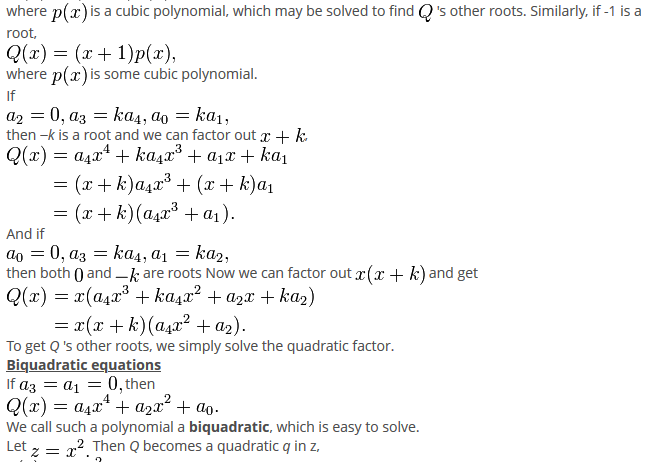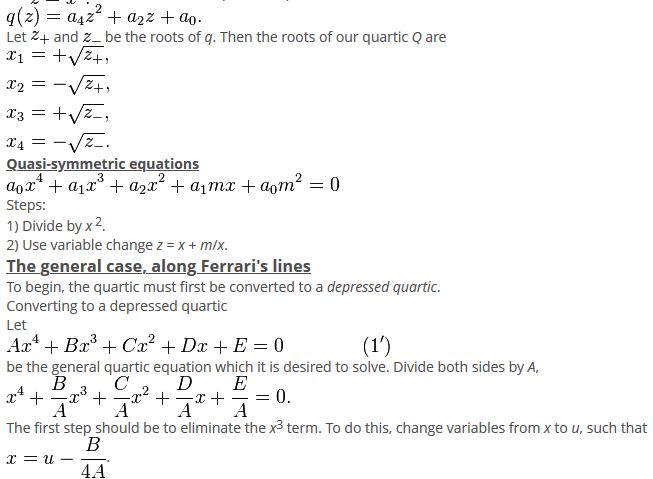×#### Thank you for registering.

One of our academic counsellors will contact you within 1 working day.

Click to Chat

1800-1023-196

+91-120-4616500

CART 0

• 0

MY CART (5)

Use Coupon: CART20 and get 20% off on all online Study Material

ITEM
DETAILS
MRP
DISCOUNT
FINAL PRICE
Total Price: Rs.

There are no items in this cart.
Continue Shopping

I wonder how could I solve a biquadratic equations of general structure "ax^4+bx^3+cx^2+dx+e" . Can any one of you pls help me.


11 years ago

							Solving a quartic equation
Special cases
Consider the quartic$Q(x) = a_4x^4+a_3x^3+a_2x^2+a_1x+a_0.\,$
If$a_0=0,\,$
then$Q(0) = 0\,$,
so zero is a root. To find the other roots, we can divide by$x\,$ and solve the resultin cubic equation$a_4x^3+a_3x^2+a_2x+a_1=0.\,$
Evident roots: 1 and −1 and −k
If$a_0+a_1+a_2+a_3+a_4=0,\,$
then$Q(1) = 0\,$,
so 1 is a root. Similarly, if$a_4-a_3+a_2-a_1+a_0=0,\,$
that is,$a_0+a_2+a_4=a_1+a_3,\,$
then -1 is a root.
When 1 is a root, we can divide$Q(x)\,$ by$x-1\,$
and get$Q(x) = (x - 1)p(x),\,$
where$p(x)\,$ is a cubic polynomial, which may be solved to find$Q\,$ 's other roots. Similarly, if -1 is a root,$Q(x) = (x + 1)p(x),\,$
where$p(x)\,$ is some cubic polynomial.
If$a_2 = 0, a_3 = ka_4, a_0 = ka_1,\,$
then −k is a root and we can factor out$x+k\,$,\begin{align} Q(x) &=a_4 x^4 + k a_4 x^3 + a_1 x + ka_1 \&=(x + k)a_4x^3 + (x + k)a_1 \&=(x + k)(a_4x^3 + a_1). \end{align}
And if$a_0=0, a_3=ka_4, a_1 = ka_2,\,$
then both$0\,$ and$-k\,$ are roots Now we can factor out$x(x + k)\,$ and get\begin{align} Q(x) &=x(a_4x^3+ka_4x^2+a_2x+ka_2) \&=x(x+k)(a_4x^2+a_2). \end{align}
To get Q 's other roots, we simply solve the quadratic factor.
If$a_3=a_1=0,\,$ then$Q(x) = a_4x^4+a_2x^2+a_0.\,\!$
We call such a polynomial a biquadratic, which is easy to solve.
Let$z=x^2.\,$ Then Q becomes a quadratic q in z,$q(z) = a_4z^2+a_2z+a_0.\,\!$
Let$z_+\,$ and$z_-\,$ be the roots of q. Then the roots of our quartic Q are\begin{align} x_1&=+\sqrt{z_+}, \x_2&=-\sqrt{z_+}, \x_3&=+\sqrt{z_-}, \x_4&=-\sqrt{z_-}. \end{align}
Quasi-symmetric equations$a_0x^4+a_1x^3+a_2x^2+a_1 m x+a_0 m^2=0 \,$
Steps:
1) Divide by x 2.
2) Use variable change z = x + m/x.
The general case, along Ferrari's lines
To begin, the quartic must first be converted to a depressed quartic.
Converting to a depressed quartic
Let$A x^4 + B x^3 + C x^2 + D x + E = 0 \qquad\qquad(1')$
be the general quartic equation which it is desired to solve. Divide both sides by A,$x^4 + {B \over A} x^3 + {C \over A} x^2 + {D \over A} x + {E \over A} = 0.$
The first step should be to eliminate the x3 term. To do this, change variables from x to u, such that$x = u - {B \over 4 A}$.
Then$\left( u - {B \over 4 A} \right)^4 + {B \over A} \left( u - {B \over 4 A} \right)^3 + {C \over A} \left( u - {B \over 4 A} \right)^2 + {D \over A} \left( u - {B \over 4 A} \right) + {E \over A} = 0.$
Expanding the powers of the binomials produces$\left( u^4 - {B \over A} u^3 + {6 u^2 B^2 \over 16 A^2} - {4 u B^3 \over 64 A^3} + {B^4 \over 256 A^4} \right) + {B \over A} \left( u^3 - {3 u^2 B \over 4 A} + {3 u B^2 \over 16 A^2} - {B^3 \over 64 A^3} \right) + {C \over A} \left( u^2 - {u B \over 2 A} + {B^2 \over 16 A^2} \right) + {D \over A} \left( u - {B \over 4 A} \right) + {E \over A} = 0.$
Collecting the same powers of u yields$u^4 + \left( {-3 B^2 \over 8 A^2} + {C \over A} \right) u^2 + \left( {B^3 \over 8 A^3} - {B C \over 2 A^2} + {D \over A} \right) u + \left( {-3 B^4 \over 256 A^4} + {C B^2 \over 16 A^3} - {B D \over 4 A^2} + {E \over A} \right) = 0.$
Now rename the coefficients of u. Let\begin{align} \alpha & = {-3 B^2 \over 8 A^2} + {C \over A} ,\\beta & = {B^3 \over 8 A^3} - {B C \over 2 A^2} + {D \over A} ,\\gamma & = {-3 B^4 \over 256 A^4} + {C B^2 \over 16 A^3} - {B D \over 4 A^2} + {E \over A} . \end{align}
The resulting equation is$u^4 + \alpha u^2 + \beta u + \gamma = 0 \qquad \qquad (1)$
which is a depressed quartic equation.
If$\beta=0 \$ then we have a biquadratic Equation, which (as explained above) is easily solved; using reverse substitution we can find our values for x.
If$\gamma=0 \$ then one of the roots is$u=0 \$ and the other roots can be found by dividing by u, and solving the resulting equation,$u^3 + \alpha u + \beta = 0 \,.$
Using reverse substitution we can find our values for x.
Regards,
Rajat,

11 years ago
							Dear Askiitians Expert, Many thanks for this method, I found very interesting. I belive that it could be generelized to higher degree equations too.

3 years ago
							Dear Student,Please find below the solution to your problem.Thanks and Regards

2 months ago
Think You Can Provide A Better Answer ?

## Other Related Questions on Algebra

View all Questions »### Course Features

• 731 Video Lectures
• Revision Notes
• Previous Year Papers
• Mind Map
• Study Planner
• NCERT Solutions
• Discussion Forum
• Test paper with Video Solution### Course Features

• 101 Video Lectures
• Revision Notes
• Test paper with Video Solution
• Mind Map
• Study Planner
• NCERT Solutions
• Discussion Forum
• Previous Year Exam Questions